# Stream and Accelerate System Simulation

Phased Array System Toolbox™ can be used to model an end-to-end phased array system - generate a transmitted waveform, simulate the target return, and then process the received signal to detect the target. This is shown in the examples Simulating Test Signals for a Radar Receiver and Waveform Design to Improve Range Performance of an Existing System. This example shows how to simulate such a system in streaming mode so you can run the simulation for a long time and observe the system dynamics.

### Simulation Setup

First, set up the radar system with some basic parameters. The entire radar system is similar to the one shown in the Waveform Design to Improve Range Performance of an Existing System example.

```fs = 6e6; bw = 3e6; c = 3e8; fc = 10e9; prf = 18750; num_pulse_int = 10; [waveform,transmitter,radiator,collector,receiver,sensormotion,... target,tgtmotion,channel,matchedfilter,tvg,threshold] = ... helperRadarStreamExampleSystemSetup(fs,bw,prf,fc,c); ```

### System Simulation

Next, run the simulation for 100 pulses. During this simulation, four time-scopes are used to observe the signals at various stages. The first three scopes display the transmitted signal, received signal, and the post-matched-filter and gain-adjusted signal for 10 pulses. Although the transmitted signal is a high-power pulse train, scope 2 shows a much weaker received signal due to propagation loss. This signal cannot be detected using the preset detection threshold. Even after matched-filtering and gain compensation, it is still challenging to detect all three targets.

```% pre-allocation fast_time_grid = 0:1/fs:1/prf-1/fs; num_pulse_samples = numel(fast_time_grid); rx_pulses = complex(zeros(num_pulse_samples,num_pulse_int)); mf_pulses = complex(zeros(num_pulse_samples,num_pulse_int)); detect_pulse = zeros(num_pulse_samples,1); % simulation loop for m = 1:10*num_pulse_int % Update sensor and target positions [sensorpos,sensorvel] = sensormotion(1/prf); [tgtpos,tgtvel] = tgtmotion(1/prf); % Calculate the target angles as seen by the sensor [tgtrng,tgtang] = rangeangle(tgtpos,sensorpos); % Simulate propagation of pulse in direction of targets pulse = waveform(); [pulse,txstatus] = transmitter(pulse); txsig = radiator(pulse,tgtang); txsig = channel(txsig,sensorpos,tgtpos,sensorvel,tgtvel); % Reflect pulse off of targets tgtsig = target(txsig); % Receive target returns at sensor rxsig = collector(tgtsig,tgtang); nn = mod(m-1,num_pulse_int)+1; rx_pulses(:,nn) = receiver(rxsig,~(txstatus>0)); % Detection processing mf_pulses(:,nn) = matchedfilter(rx_pulses(:,nn)); mf_pulses(:,nn) = tvg(mf_pulses(:,nn)); % Perform pulse integration every 'num_pulse_int' pulses if nn == num_pulse_int detect_pulse = pulsint(mf_pulses,'noncoherent'); end helperRadarStreamDisplay(pulse,abs(rx_pulses(:,nn)),... abs(mf_pulses(:,nn)),detect_pulse,... sqrt(threshold)*ones(num_pulse_samples,1)); end ```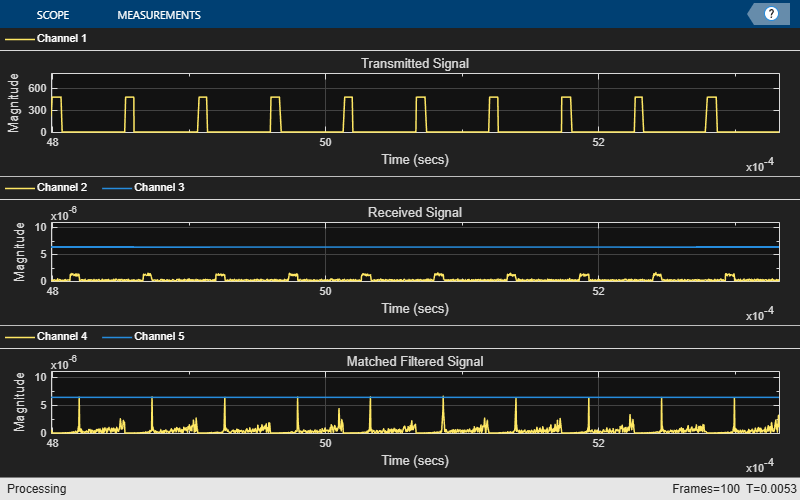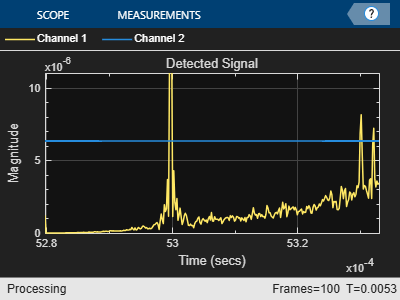### Improve Simulation Speed Using Code Generation

Because radar systems require intensive processing, simulation speed is a major concern. After you have run 100 pulse to check out your code, you may want to run 1000 pulses. When you run the simulation in interpreted MATLAB® mode, you can measure the elapsed time using:

```tic; helperRadarStreamRun; time_interpreted = toc ```
```time_interpreted = 8.0410 ```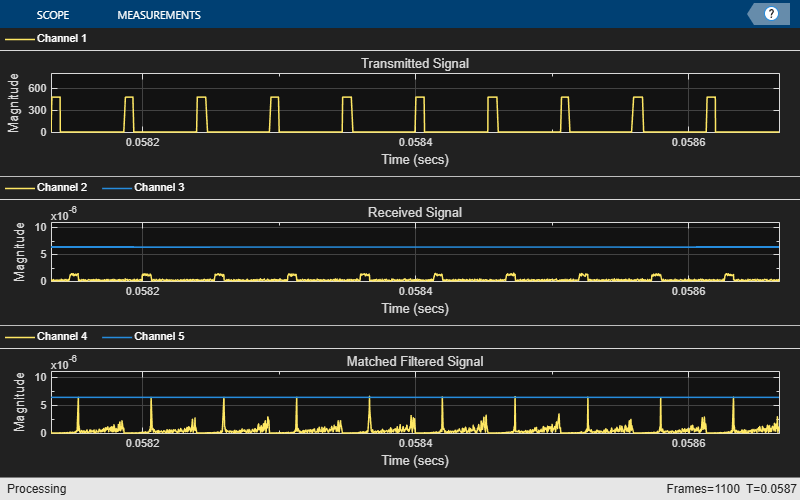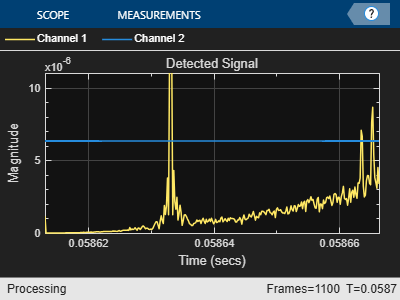If the simulation is too slow, you can speed it up using MATLAB Coder™. MATLAB Coder can generate compiled MATLAB code resulting in significant improvement in processing speed. In this example, MATLAB Coder generates a helperRadarStreamRun_mex function from the helperRadarStreamRun function.

```codegen helperRadarStreamRun.m ```
```Code generation successful. ```

When the mex version is invoked, the simulation speed is improved.

```tic; helperRadarStreamRun_mex; time_compiled = toc ```
```time_compiled = 2.0587 ```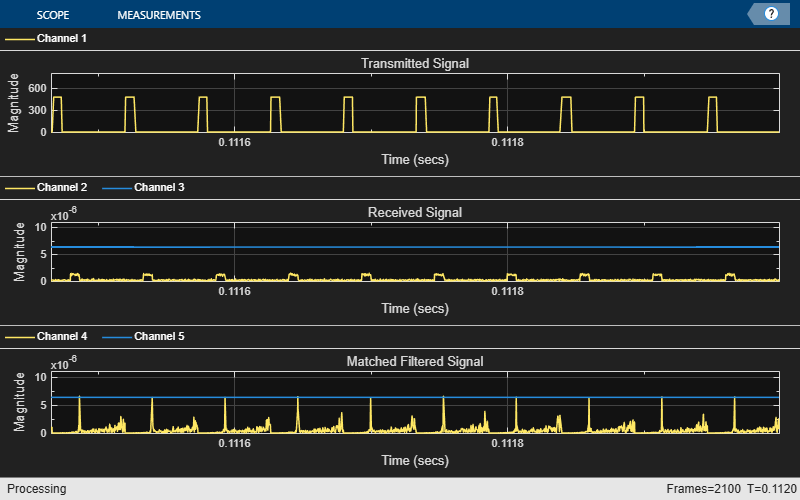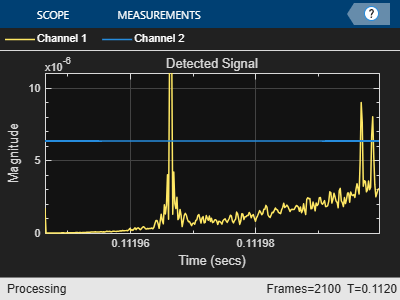Speedup improvement depends on several factors such as machine CPU speed and available memory but is typically increased 3-4 times. Note that the visualization of data using scopes is not sped up by MATLAB Coder and is still handled by the MATLAB interpreter. If visualizations are not critical to your simulation, then you can remove them for further speed improvement.

1. The visualization capability in generated code is very limited compared to what is available in MATLAB. If you need to keep the visualization in the simulation, use the `coder.extrinsic` trick; but this slows down the simulation.